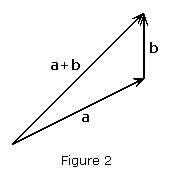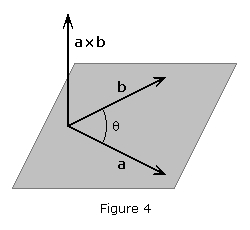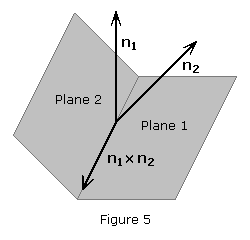## VectorsThis is a basic introduction to the mathematics of vectors. Vectors are defined as mathematical expressions possessing magnitude and direction, which add according to the parallelogram law. Forces, displacements, velocities, accelerations, and momenta are examples of physical quantities that may be represented mathematically by vectors. Physical quantities that do not have direction, such as volume, mass or energy, are represented by ordinary number or scalars.

In this article, scalar variables will be denoted as an italicized variable, such as a. Variables that are vectors will be indicated with a boldface variable, such as a, although it is common to see vectors denoted with small arrows above the variable.

In many problems it will be found desirable to resolve a force into two or three components that are perpendicular to each other. These component vectors are generally oriented on a coordinate system, the most popular of which is the two-dimensional Cartesian plane. The Cartesian plane has a horizontal axis which is labeled x and a vertical axis labeled y. One can use the same principle to specify the position of any point in three-dimensional space by three Cartesian coordinates, in which the axes are x, y, and z.

Vectors in multiple-dimension coordinate systems can be resolved into their component vectors. In the three-dimensional case, this results in an x-component, a y-componentand a z-component. Figure 1 to the right is an example of a vector, a, resolved into its components, axay and az. When breaking a vector into its components, the vector is a sum of the components,

a = ax + ay + azAdding scalar quantities ignores all information about the directions; however, vectors are manipulated somewhat differently – the direction must always be considered. Adding two or more vectors is synonymous to placing the vectors end to end, and creating a new vector running from the starting point to the end point, as demonstrated in Figure 2. If the vectors have the same direction, then this simply means adding the magnitudes, but if they have different directions, the addition is more complex. Vectors are added by resolving them into their components and then adding the components, as shown below,

a + b = (ax + bx) + (ay + by) + (az + bz)

The subtraction of a vector is defined as the addition of the corresponding negative vector, that is, by a – b we mean a + (–b).

a – b = (ax – bx) + (ay – by) + (az – bz)

Scalar Multiplication

The operation of multiplying a vector by a scalar is called scalar multiplication. If we define the product na of a scalar n and a vector a as a vector having the same direction as a (if n positive), or a direction opposite to that of a (if n negative), and a magnitude equal to the product of a and of the absolute value of n. The resulting vector is:

n a = n ax + n ay + n az

Scalar multiplication is distributive over vector addition in the following sense:

n (a + b) = n a + n b

Unit Vectors

We shall, at this point, introduce three vectors of magnitude 1, directed respectively along the positive x-, y- and z-axes. These vectors are called unit vectors and are denoted by ij and k, respectively. Recalling the definition of the product of a scalar and a vector, we note that the rectangular components axay and az of a vector a may be obtained by multiplying respectively the unit vectors ij and k by appropriate scalars. We write

a = ax i + ay j + az k

While the scalars axay and az may be positive or negative, depending on the sense of axay and az, their absolute values are respectively equal to the magnitudes of the component vectors axay and az. The scalarsaxay and az are called the scalar components of the vector a, while the actual components axay and az should be referred to as the vector components of a. However, when there exists no possibility of confusion, the vector as well as the scalar components of a may be referred to simply as the components of a. We note that the scalar component of ax is positive when the vector component ax has the same sense as the unit vector i (i.e. the same sense as the positive x-axis) and negative when ax has the opposite sense. A similar conclusion may be drawn regarding the sign of the scalar components ay and az.

Magnitude

The magnitude or length of the vector a, represented a = |a|, may be obtained by applying the Pythagorean theorem

a = ( ax2 + ay2 + az2 ) 1/2

Dot ProductThe dot product of two vectors a and b is defined as the product of the magnitudes of a and b and the cosine of the angleformed by a and b. The dot product of a and b is denoted by a • b and is defined as:

a • b = a b cosNote that the expression just defined is not a vector, but a scalar, which explains why the dot product is also referred to as the scalar product of a and b.

The dot product can also be defined as the sum of the products of the components of each vector,

a • b = axbx + ayby + azbz

Combining the above equations, we see that

cos= (axbx + ayby + azbz) / (a b)

Cross ProductThe cross product of two vectors a and b, denoted a × b, is defined as the vector that satisfies the following conditions:

The line of action of a × b is perpendicular to the plane containing a and b (see Figure 4).

• The magnitude of a × b is the product of the magnitudes of a and b and the sine of the angleformed by a and b.
• The sense of a × b is such that a person located at the tip of a × b will observe as counterclockwise the rotation throughthat brings a in line with b. The three vectors ab and a × b – taken in that order – are said to form a right-handed triad.

The cross product differs from the dot product primarily in that the result of the cross product of two vectors is a vector, thus it is also referred to as the vector product. The cross product is defined as

a × b = a b sin(n

where n is a unit vector perpendicular to both a and b. The cross product can also be written as

a × b = (aybz – azbyi + (azbx – axbzj + (axby – aybxk

Line of Intersection between two PlanesIf we are given two vectors, n1 and n2, that are normal to Plane 1 and Plane 2, then by simple geometrical reasoning, the line of intersection of the two planes is perpendicular to both normals (see Figure 5). To find the position vector, r, of any point on the line of intersection, we (1) find a vector, v, to which the line is parallel, and (2) find the position vector, a, of a specific point on the line. As we found above, the cross product of two vectors gives a third vector that is perpendicular to both vectors. We know that v is perpendicular to both n1 and n2; therefore,v is parallel to n1 × n2. To find a, we find any point on the line of intersection that satisfies the equations of both planes. If we let the point have an x-coordinate of zero, then we have two equations in two unknowns, y and z. Solving for y and z and setting x = 0, we have a point on the line of intersection who’s position vector is a. Substituting v and a into the following yields an equation for the line of intersection:

r = a + t v

Transformation of Coordinates

In astrodynamics it is common to give position vectors in polar coordinates of longitude, l, latitude, b, and radial distance, r. These coordinates are converted to XYZ rectangular coordinates by the following formulae:

x = r cos b cos l
y = r cos b sin l
z = r sin b

Given XYZ coordinates, longitude and latitude are derived as follows:

l = arctan[ y / x ]
b = arctan[ z / (x2 + y2)1/2 ] = asin[ z / r ]

where r is simply the vector magnitude. It is important that special care be taken to place l in the correct quadrant.

This entry was posted in Various and tagged , . Bookmark the permalink.